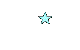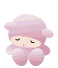~INDIRECT PROOF~

*~Indirect Proof~*Indirect Proofis when you are using proofs that are incorrect or not possible to conclude to a consumption.To use indirect proof, you must follow the 4 steps.
1. State that the result to be proved is either true or false.
2. Assume that the result to be proved is false.
3. Show that a conclusion can be reached that contradicts the known facts.
4. Since there is a contradiction, the assumption in step 2. is incorrect. Using step 1., we conclude that the result to be proved is true.

Example
In triangle ABC, M is a point on BC such that BM is not equal to CM and AM bisects angle A. Prove that AB is not Equal to AC.Using indirect proof.
Step 1) Either AB is not equal to AC or AB is equal to AC
Step 2) Assume that AB equals AC
Step 3) In triangle ABM and triangle ACM,

AB=AC
angle BAM=angle CAM
AM=AM
Therefore, triangle ABM is congruent to triangle ACM (SAS)

Since the triangles are congruent, BM= CM
This contradicts the given information that BM is not equal to CM.

Step 4) The assumptin that AB = AC is incorrect. Therefore, AB is not equal to AC.